﻿ 基于C#语言的国(境)外地震背景信息交互式产出系统文章快速检索
 地震地磁观测与研究2021, Vol. 42Issue (1): 162-168  DOI: 10.3969/j.issn.1003-3246.2021.01.0220

### 引用本文MA Xiudan, CHEN Shuqiao, LONG Haiyun, et al. The design and implementation of the interactive output system of overseas Earthquake background information based on C#[J]. Seismological and Geomagnetic Observation and Research, 2021, 42(1): 162-168. DOI: 10.3969/j.issn.1003-3246.2021.01.022.### 文章历史

The design and implementation of the interactive output system of overseas Earthquake background information based on C#
MA Xiudan, CHEN Shuqiao , LONG Haiyun , ZHANG Jinhui
China Earthquake Networks Center, Beijing 100045, China
Abstract: A new interactive output system of overseas Earthquake background information was designed. This system is developed by C# language. It solves the problems encountered in the generation of Earthquake special reports, such as information source, standardization, and timeliness. With a little personnel participation, the system can automatically obtain the population information, tsunami information, historical earthquake catalogue within a certain range of epicenter and generate the estimation information of casualties in an earthquake. Subsequently, it produces the earthquake special report in word format. The system can quickly produce the first-period earthquake report, which provides support for all units to make response strategies in time.
Key words: earthquake background information    C# programming language    interactive    historical earthquake catalogue
0 引言

1 地震背景信息

2 系统设计与实现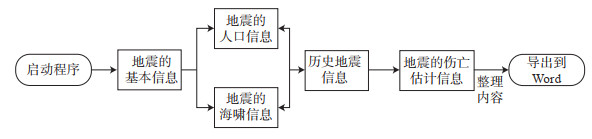图 1 国(境)外地震背景信息生成流程 Fig.1 Flow chart of overseas Earthquake background information generation

2.1 地震基本信息的获取

HtmlWeb htmlWeb = new HtmlWeb(); //

HtmlNode region_infor = doc.DocumentNode.SelectNodes(".//div[@id='speed-search']");

//获取id='speed-search'属性的第一个div节点，得到网页中包含地震信息的模块

HtmlNodeCollection hrefList = region_infor.SelectNodes(".//tr"); //获取tr节点的集合，得到多个地震信息

List < string> pageHtml = new List < string>(); //新建一个string类型的列表。用于存放地震的经纬度、时间、震级、深度、地点等。

//循环获取地震的基本信息，包括时间、经纬度、震级、深度、地点等存放到pageHtml列表中。

foreach (HtmlNode href in hrefList)

{

string att = href.InnerText;

}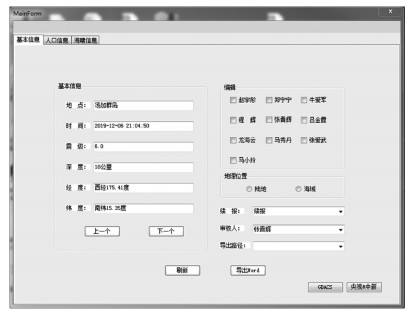图 2 国(境)外地震背景信息交互产出系统主界面 Fig.2 The main interface of the system
2.2 地震人口信息的获取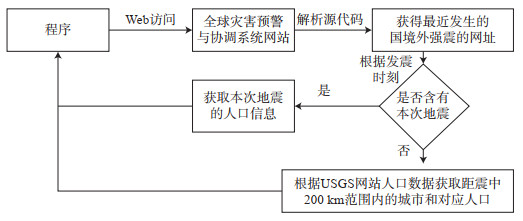图 3 人口信息获取流程 Fig.3 Flow chart of population information acquisition
2.3 地震海啸信息的获取

2.4 地震人员伤亡信息的获取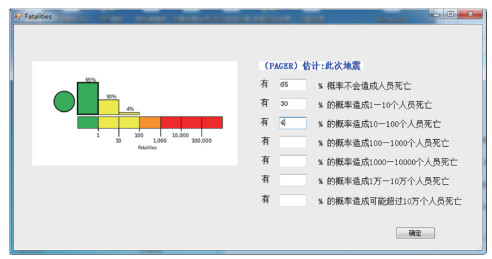图 4 地震人员伤亡信息的界面 Fig.4 Interface for inputting casualty information
2.5 历史地震信息的获取

(1) 采用角度转弧度公式分别将震中的经度和纬度转换成弧度

 $\begin{array}{l} 弧度 = 度数 \cdot \left({{\rm{ \mathsf{ π} }}/180} \right)\\ {\rm{lat}}{{\rm{1}}_弧 } = {\rm{lat1}} \cdot \left({{\rm{ \mathsf{ π} }}/180} \right)\\ {\rm{lon}}{{\rm{1}}_弧 } = {\rm{lon1}} \cdot \left({{\rm{ \mathsf{ π} }}/180} \right)\\ {\rm{lat}}{{\rm{2}}_弧 } = {\rm{lat2}} \cdot \left({{\rm{ \mathsf{ π} }}/180} \right)\\ {\rm{lon}}{{\rm{2}}_弧 } = {\rm{lon2}} \cdot \left({{\rm{ \mathsf{ π} }}/180} \right) \end{array}$ (1)

(2) 计算震中A和震中B经纬度差值的绝对值

 $\begin{array}{l} {\rm{lo}}{{\rm{n}}_弧 } = \left| {{\rm{lon}}{{\rm{l}}_弧 } - {\rm{lon}}{{\rm{2}}_弧 }} \right|\\ {\rm{la}}{{\rm{t}}_弧 } = \left| {{\rm{lat}}{{\rm{l}}_弧 } - {\rm{lat}}{{\rm{2}}_弧 }} \right| \end{array}$ (2)

(3) 采用Haversine公式计算∠AOB的弧度数，O为地球的球心。

 $\begin{array}{l} \;\;\;\;\;\;\;\;\;\;\;\;\;\;\;\;\;\;\;\;\;\;\;\;\;\;\;\;\;\;\;\;\;\;{\rm{haver}}\;{\rm{sin}}\theta {\rm{ = si}}{{\rm{n}}^2}\left({\theta /2} \right) = \left({1 - \cos \theta } \right)/2\\ \angle {\rm{AO}}{{\rm{B}}_弧 } = 2 \times {\rm{arcsin}}\sqrt {{\rm{haver}}\;{\rm{sin}}\left({{\rm{la}}{{\rm{t}}_差 }} \right) + \cos \left({{\rm{lat}}{{\rm{1}}_弧 }} \right) \times \cos \left({{\rm{lat}}{{\rm{2}}_弧 }} \right) \times {\rm{haver}}\;{\rm{sin}}\left({{\rm{lo}}{{\rm{n}}_差 }} \right)} \end{array}$ (3)

(4) 根据弧长的计算公式，代入地球半径计算2点之间在球面的距离

 $\begin{array}{l} 弧长 = 圆心角弧度数 \times 半径 \\ 震中距 = \angle {\rm{AO}}{{\rm{B}}_弧 } \times R \end{array}$ (4)

R为地球半径，这里取平均值6 371 km。

(5) 通过统计一定范围内地震的震级，计算出每个震级范围内的历史地震数量以及时间最近、空间最近和震级最大的历史地震信息。

3 效果展示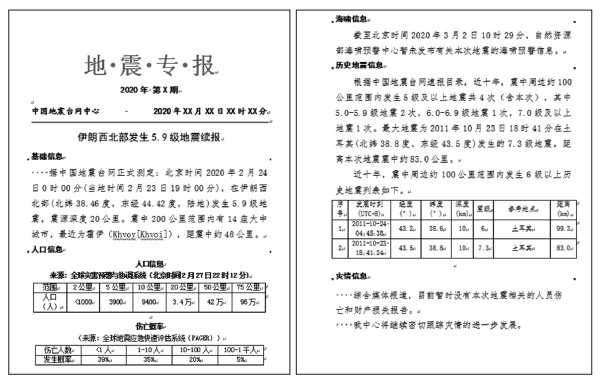图 5 地震专报样例 Fig.5 Example of an earthquake special report
4 结束语

 常亮, 郭垚嘉, 张幸兴, 等. 基于Android系统的测震台网日志管理平台设计[J]. 地震地磁观测与研究, 2019, 40(4): 160-166. 高峰, 杨学山, 马树林. 地震预警系统综述[J]. 自然灾害学报, 2014, 23(5): 62-69. 姜立新, 帅向华, 张建福, 等. 地震应急指挥管理信息系统的探讨[J]. 地震, 2003, 23(2): 115-120. 李宇航, 张军华, 龚辛侬, 等. 基于C#的三维可视化显示系统编程及实现[C]//2014年中国地球科学联合学术年会——专题19: 地震波传播与成像论文集. 北京: 中国地球物理学会, 中国地质学会, 2014. 梁艳, 高云峰. 基于Java的地震应急流动测震及强震动自动化产出系统[J]. 地震地磁观测与研究, 2019, 40(4): 146-154. 刘颖, 庹先国, 刘勇. 基于C#.NET的地震数据采集终端软件设计和实现[J]. 自动化仪表, 2017, 38(10): 74-77. 孙振凯. PAGER——地震影响的快速评估(USGS情况说明书第2010-3036号)[J]. 国际地震动态, 2013(1): 4-7. 陶鑫, 肖健, 李雨泽, 等. 基于c#的全球地震速报信息接收系统的设计与实现[J]. 科技展望, 2017, 27(2): 25. 韦东. 浅谈C#语言基础[J]. 计算机光盘软件与应用, 2011(8): 218. 杨伯舫. 解放生产力的关键是解放劳动力[J]. 现代哲学, 1993(2): 6-10. 赵纪东, 张志强. 地震预警系统的发展、应用及启示[J]. 地质通报, 2009, 28(4): 456-462. 赵志远, 宋昭, 张波, 等. 基于Matlab的一维电测深正反演可视化软件设计[J]. 地震地磁观测与研究, 2019, 40(4): 155-159. 朱涛. 基于C#. NET的远程智能管理系统设计与实现[D]. 北京: 北京邮电大学, 2015.